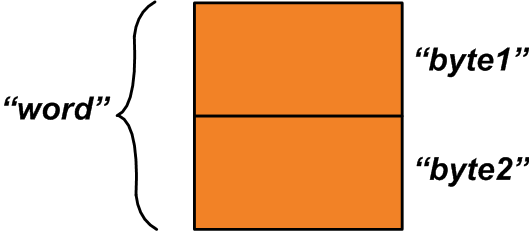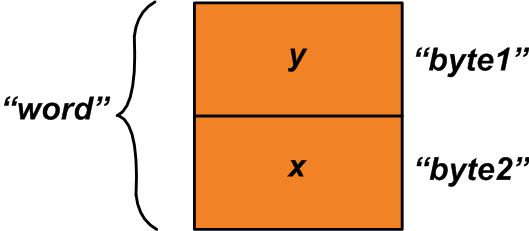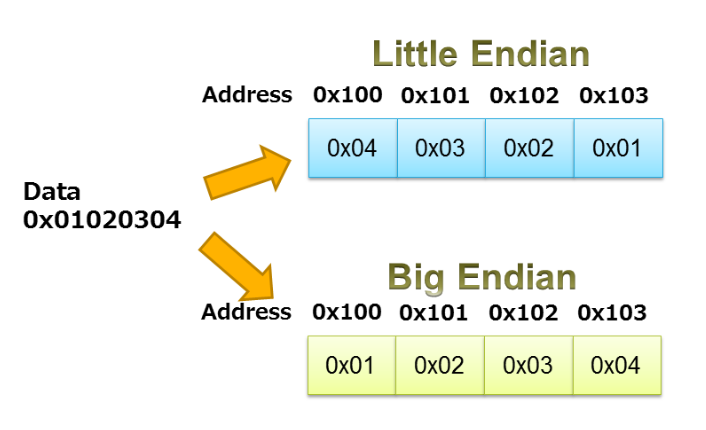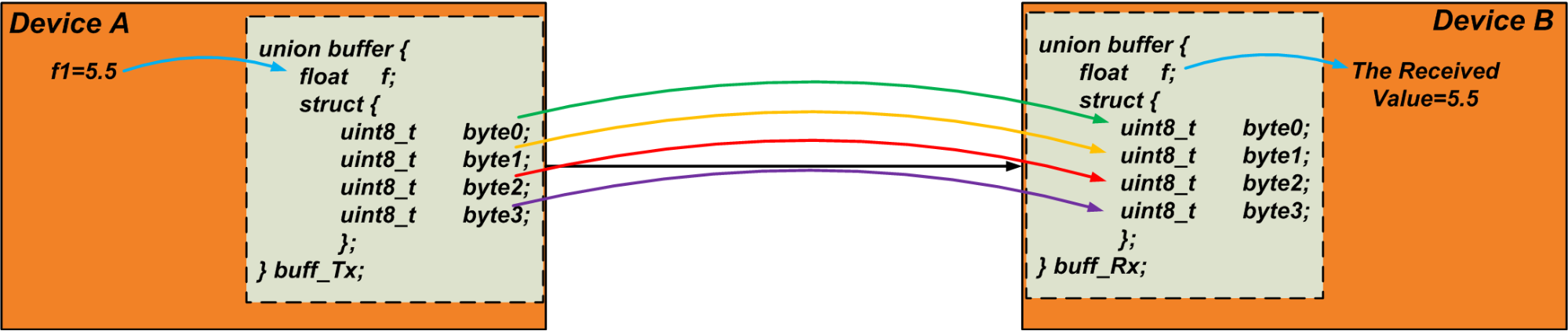Technical Article

# Union in C Language for Packing and Unpacking Data

June 05, 2019 by Dr. Steve Arar

## Learn about packing and unpacking data with unions in C language.

Learn about packing and unpacking data with unions in C language.

In a previous article, we discussed that the original application of unions had been creating a shared memory area for mutually exclusive variables. However, over the time, the programmers have widely used unions for a completely different application: extracting smaller parts of data from a larger data object. In this article, we’ll look at this particular application of unions in greater detail.

### Using Unions For Packing/Unpacking Data

The members of a union are stored in a shared memory area. This is the key feature that allows us to find interesting applications for unions.

Consider the union below:

union {
uint16_t   word;
struct {
uint8_t   byte1;
uint8_t   byte2;
};
} u1;

There are two members inside this union: The first member, “word”, is a two-byte variable. The second member is a structure of two one-byte variables. The two bytes allocated for the union is shared between its two members.

The allocated memory space can be as shown in Figure 1 below.##### Figure 1

While the “word” variable refers to the whole allocated memory space, the “byte1” and “byte2” variables refer to the one-byte areas that construct the “word” variable. How can we use this feature? Assume that you have two one-byte variables, “x” and “y”, that should be combined to produce a single two-byte variable.

In this case, you can use the above union and assign “x” and “y” to the structure members as follows:

u1.byte1 = y;
u1.byte2 = x;

Now, we can read the “word” member of the union to get a two-byte variable composed of “x” and “y” variables (See Figure 2).##### Figure 2

The above example shows the use of unions for packing two one-byte variables into a single two-byte variable. We could also do the reverse: write a two-byte value to “word” and unpack it into two one-byte variables by reading the “x” and “y” variables. Writing a value to one member of a union and reading another member of it is sometimes referred to as “data punning”.

### The Processor Endianness

When using unions for packing/unpacking data, we need to be careful about the processor endianness. As is discussed in Robert Keim's article on endianness, this term specifies the order in which the bytes of a data object are stored in memory. A processor can be little endian or big endian. With a big-endian processor, data is stored in a way that the byte containing the most significant bit has the lowest memory address. In little-endian systems the byte containing the least significant bit is stored first.

The example depicted in Figure 3 illustrates the little endian and big endian storage of the sequence 0x01020304.##### Figure 3. Image courtesy of IAR.

Let’s use the following code to experiment with the union of the previous section:

#include <stdio.h>
#include <stdint.h>

int main()
{

union {
struct{
uint8_t   byte1;
uint8_t   byte2;
};
uint16_t    word;

} u1;

u1.byte1 = 0x21;
u1.byte2 = 0x43;

printf("Word is: %#X", u1.word);
return 0;
}

Running this code, I get the following output:

Word is: 0X4321

This shows that the first byte of the shared memory space (“u1.byte1”) is used to store the least significant byte (0X21) of the “word” variable. In other words, the processor I’m using to execute the code is little endian.

As you can see, this particular application of unions can exhibit implementation-dependent behavior. However, this shouldn’t be a serious problem because for such low-level coding, we usually know the endianness of the processor. In case we don’t know these details, we can use the above code to find out how the data is organized in the memory.

### Alternative Solution

Instead of using unions, we can also use the bitwise operators to perform data packing or unpacking. For example, we can use the following code to combine two one-byte variables, “byte3” and “byte4”, and produce a single two-byte variable (“word2”):

word2 = (((uint16_t)   byte3) << 8 ) | ((uint16_t)   byte4);

Let’s compare the output of these two solutions in the little endian and big endian cases. Consider the code below:

#include <stdio.h>
#include <stdint.h>

int main()
{
union {
struct {
uint8_t   byte1;
uint8_t   byte2;
};
uint16_t    word1;
} u1;

u1.byte1 = 0x21;
u1.byte2 = 0x43;
printf("Word1 is: %#X\n", u1.word1);

uint8_t       byte3, byte4;
uint16_t      word2;
byte3 = 0x21;
byte4 = 0x43;
word2 = (((uint16_t) byte3) << 8 ) | ((uint16_t) byte4);
printf("Word2 is: %#X \n", word2);

return 0;
}

If we compile this code for a big endian processor such as TMS470MF03107, the output will be:

Word1 is: 0X2143

Word2 is: 0X2143

However, if we compile it for a little endian processor such as STM32F407IE, the output will be:

Word1 is: 0X4321

Word2 is: 0X2143

While the union based method exhibits hardware dependent behavior, the method based on the shift operation leads to the same result regardless of the processor endianness. This is due to the fact that, with the latter approach, we are assigning a value to the name of a variable (“word2”) and the compiler takes care of the memory organization employed by the device. However, with the union based method, we are changing the value of the bytes that construct the “word1” variable.

Although the union based method exhibits hardware dependent behavior, it has the advantage of being more readable and maintainable. That’s why many programmers prefer to use unions for this application.

### A Practical Example of “Data Punning”

When working with common serial communication protocols, we may need to perform data packing or unpacking. Consider a serial communication protocol that sends/receives one byte of data during each communication sequence. As long as we’re working with one-byte long variables, it’s easy to transfer the data but what if we have a structure of arbitrary size that should go through the communication link? In this case, we have to somehow represent our data object as an array of one-byte long variables. Once we get this array-of-bytes representation, we can transfer the bytes through the communication link. Then, in the receiver end, we can pack them appropriately and rebuild the original structure.

For example, assume that we need to send a float variable, “f1”, through the UART communication. A float variable usually occupies four bytes. Therefore, we can use the following union as a buffer for extracting the four bytes of “f1”:

union {
float    f;
struct {
uint8_t   byte;
};
} u1;

The transmitter writes the variable “f1” to the float member of the union. Then, it reads the “byte” array and sends the bytes down the communication link. The receiver does the reverse: it writes the received data to the “byte” array of its own union and reads the float variable of the union as the received value. We could do this technique to transfer a data object of arbitrary size. The following code can be a simple test for verifying this technique.

#include <stdio.h>
#include <stdint.h>

int main()
{

float f1=5.5;

union buffer {
float f;
struct {
uint8_t byte;
};
};

union buffer buff_Tx;
union buffer buff_Rx;
buff_Tx.f = f1;
buff_Rx.byte = buff_Tx.byte;
buff_Rx.byte = buff_Tx.byte;
buff_Rx.byte = buff_Tx.byte;
buff_Rx.byte = buff_Tx.byte;

printf("The received data is: %f", buff_Rx.f);

return 0;
}

Figure 4 below visualizes the discussed technique. Note that the bytes are transferred sequentially.### Conclusion

While the original application of unions was creating a shared memory area for mutually exclusive variables, over the time, the programmers have widely used unions for a completely different application: using unions for data packing/unpacking. This particular application of unions involves writing a value to one member of the union and reading another member of it.

“Data punning” or using unions for data packing/unpacking can lead to hardware-dependent behavior. However, it has the advantage of being more readable and maintainable. That’s why many programmers prefer to use unions for this application. “Data punning” can be particularly helpful when we have a data object of arbitrary size that should go through a serial communication link.•Ahmet Faruk ÜNAL June 07, 2019